## Spotting Out The Embedded Figures

#### Spotting Out The Embedded Figures

1. Select the answer figure in which the question figure is hidden ?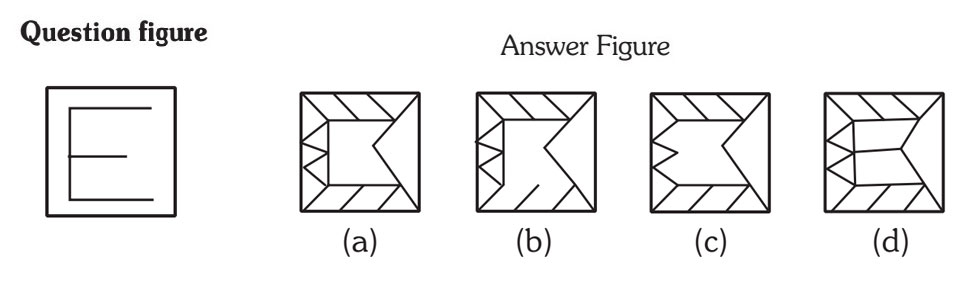1. (a)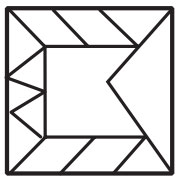2. (b)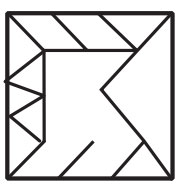3. (c).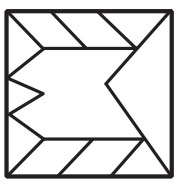4. (d).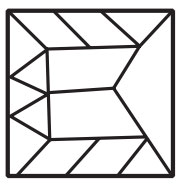1. Check the figure in which letter E available.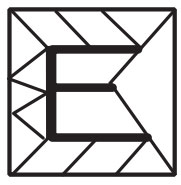##### Correct Option: D1. Select the answer figure in which the question figure is hidden ?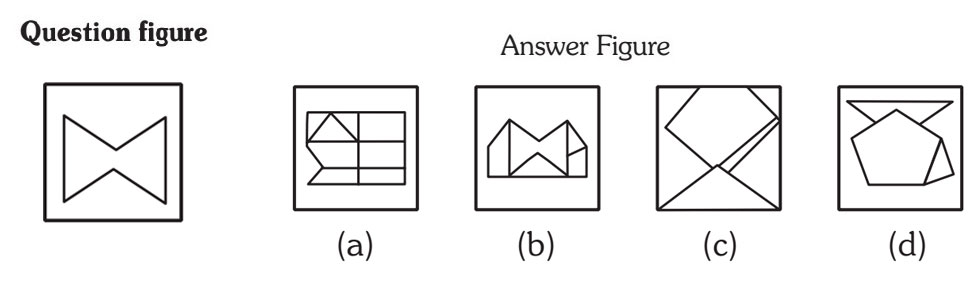1. (a)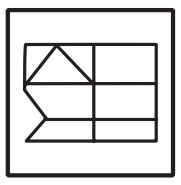2. (b).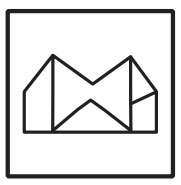3. (c).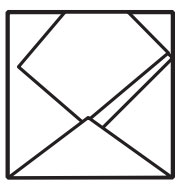4. (d).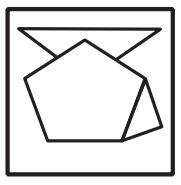1. .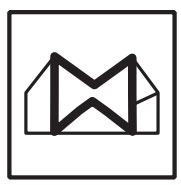##### Correct Option: B1. Select the answer figure in which the question figure is hidden ?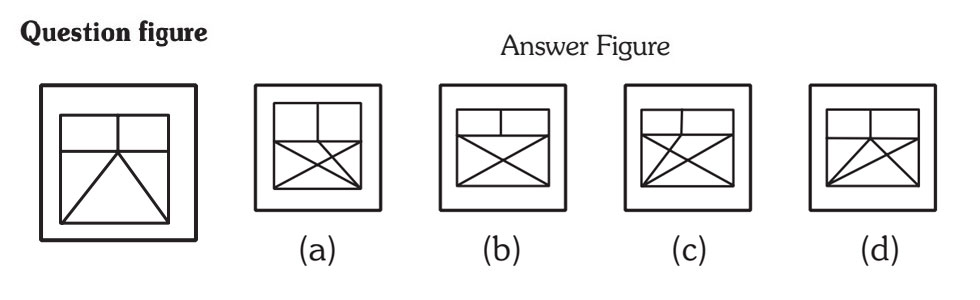1. (a)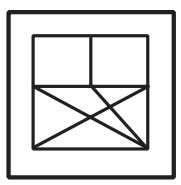2. (b).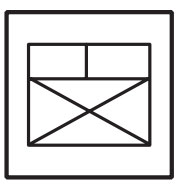3. (c).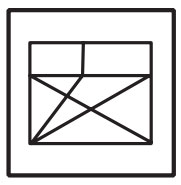4. (d).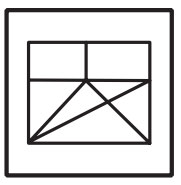1. Please check the highlighted area in given figure option.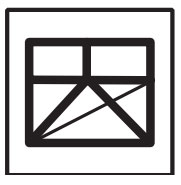##### Correct Option: D1. Select the answer figure in which the question figure is hidden ?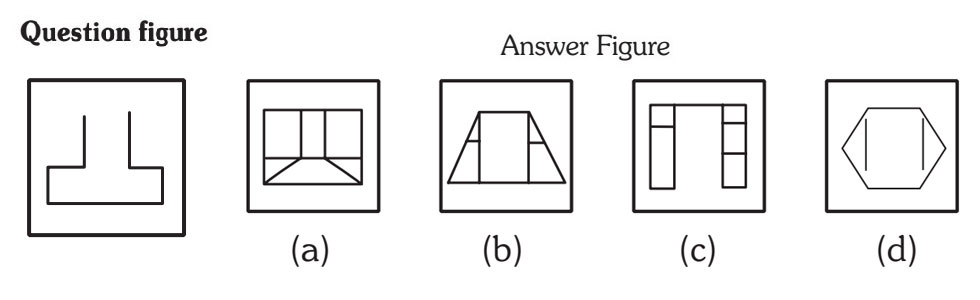1. (a)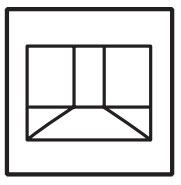2. (b).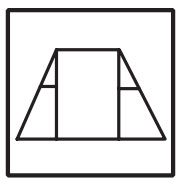3. (c).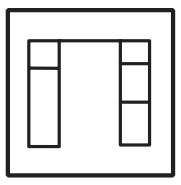4. (d).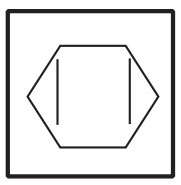1. search the highlighted part in given option figure.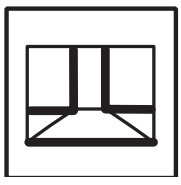##### Correct Option: A1. Select the answer figure in which the question figure is hidden ?
.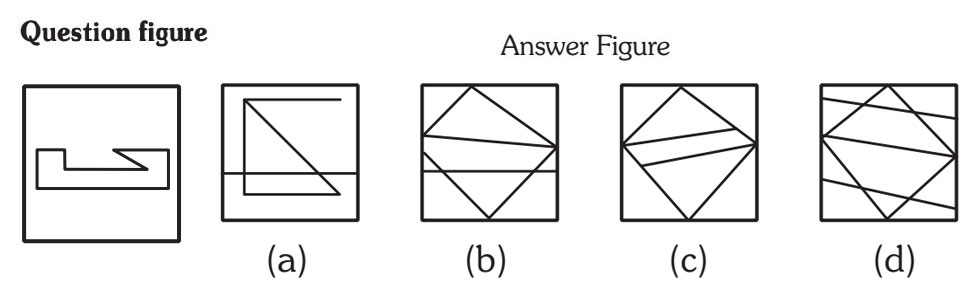1. (a)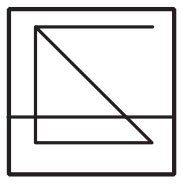2. (b).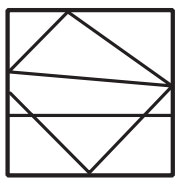3. (c).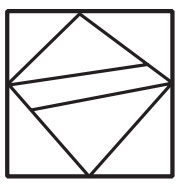4. (d).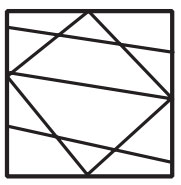1. Check the highlighted parts in given figure.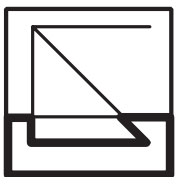##### Correct Option: A